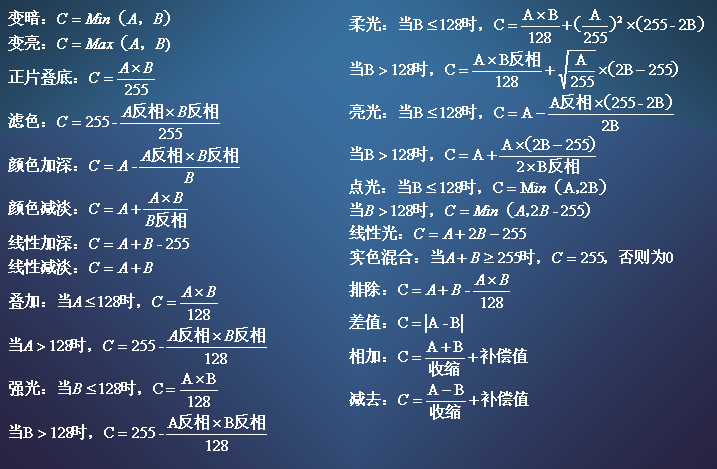A 代表下面图层的颜色值；
B 代表上面图层的颜色值；
C 代表混合图层的颜色值；
d 表示该层的透明度。

1.Opacity 不透明度

C=d×A+(1-d)×B

2.Darken 变暗

B<=A 则 C=B B>=A 则 C=A

3.Lighten 变亮

B<=A 则 C=A B>A 则 C=B

4.Multiply 正片叠底

C=(A×B)/255

5.Screen 滤色

C=255-(A反相×B反相)/255

（就好像两台投影机分别对其中一个图层进行投影后，然后投射到同一个屏幕上）。

6.Color Burn 颜色加深

C=A-(A反相×B反相)/B

7.Color Dodge 颜色减淡

C=A+(A×B)/B反相

8.Linear Burn 线形加深

C=A+B-255

9.Linear Dodge 线形减淡

C=A+B

10.Overlay叠加

A<=128 则 C=(A×B)/255 A>128 则 C=255-(A反相×B反相)/128

11.Hard Light 强光

B<=128 则 C=(A×B)/128 B>128 则 C=255-(A反相×B反相)/128

12.Soft Light柔光

B<=128 则 C=(A×B)/128+(A/255)^2×(255-2B) B>128 则 C=(A×B反相)/128+sqrt(A/255)×(2B-255)

13.Vivid Light 亮光

B<=128 则 C=A-A反相×(255-2B)/(2B) B>128 则 C=A+A×(2B-255)/(2×B反相)

14.Linear Light 线形光

C=A+2×B-255

15.Pin Light 点光

B<=128 则 C=Min (A,2B) B>128 则 C=Min(A,2B-255)

16.Hard Mix 实色混合

A+B>=255 则 C=255
A+B

17.Difference 差值

C=|A-B|

18.Exclusion 排除

C=A+B-(A×B)/128

19.Hue 色相

HcScYc =HBSAYA

20.Saturation 饱和度

HcScYc =HASBYA

21.Color 颜色

HcScYc =HBSBYA

22.Luminosity 亮度

HcScYc =HASAYB

23.Dissolve 溶解铁血 汉子 2020年5月16日
2020/07/11/01:48:01pm 2020/5/16/3:50:51
0 55